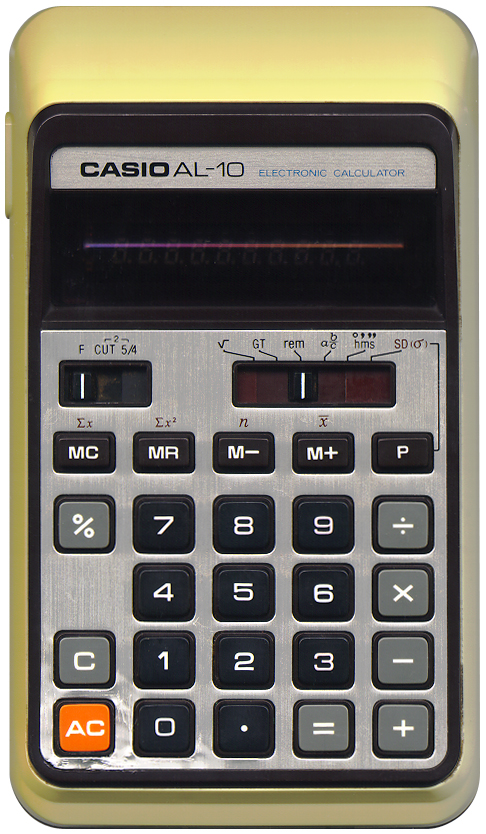# CASIO AL‑10

## Description

 Brand: CASIO Model: AL-10 Type: Almost scientific calculator Picture:Batteries: AA x 2 Lifetime: Introduced: 1975 Terminated: unknown Notes: This is a peculiar calculator. It has a special key, the "P"-key which function is determined using a slider. This slider has the following positions: √ - Square roots. The "P"-key will calculate the square root of a gven number. GT - Memory calculation. The "P"-key will display the "Grand Total" of all calculations so far. Every time the "="-key is pressed after the last time the "AC"-key was pressed, the result is added to this "Grand Total". The "Grand Total" memory works independent from the calculators regular memory. rem - Remainder This setting will change the operation of divisions. Divisions are performed as with integer results, and the "P"-key will show the remainder. Thus, when performing 6 / 4, the result will be 1, and the "P"-key will yield 2. ab/c - Fractional calculation. This will change the "P"-key into the "fraction" key present on many CASIO calculators, and will let the calculator operate on fractions. hms - Time calculation. This will change the "P"-key into the "hours minutes seconds" key present on many CASIO calculators. SD - Standard deviation. This will change some keys on the calculator. The "="-key will act as a Σ+ operator. The memory keys will perform the statistical functions displayed above them. The normal memory functions are unavailable in this mode. The "P"-key will calculate the standard deviation. All in all, a very unusual design. Even though this calculator has some sophisticated scientific functions it has a quite common bug in that this calculator has no problems with negative square roots and thinks √-9 = -3.